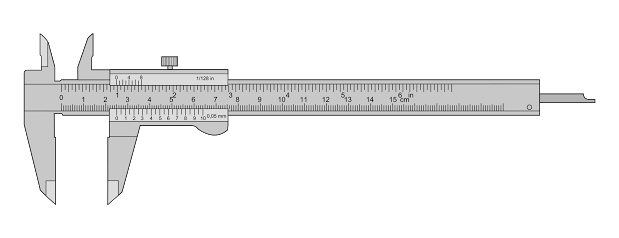# Will You Multiply The Error?The side of a cubical block when measured with a vernier caliper is $2.50\text{ cm}$. The vernier constant is $0.01\text{ cm}$. The maximum possible error in the area of one face (in $\text{cm}^2$) is

×